# Multiplication Time Table

### What is Multiplication Table? Definition and Explanation

Multiplication tables are a valuable tool that can be used in many different ways. They are commonly used in school to help students learn multiplication, and they can also be used in other areas of life, such as solving word problems or finding missing products.

In this blog, we will outline the basics of multiplication tables and how they can be used to benefit your education and everyday life.

### What is a Multiplication Table?

A multiplication table is a grid with the numbers 1 to 12 listed in the first row and column. Each subsequent row and column contains the product of the numbers in the previous row and column.

For example, if you want to find the product of 2 x 3, you would look at the first row of the table (2). Next, you would look at the second row (3), and see the intersecting point of both numbers which would be 6.

The table can be used to calculate any two numbers within its range, which makes it a valuable tool for teaching multiplication. It’s also great for students who need to remember their multiplication facts quickly.

For example, if you wanted to know what 12 x 15 is, simply look at the sixth row of the table which states that 12 multiplied by 15 equals 180. This method makes it easy for students to remember large numbers without having to memorize them.

Multiplication tables are also great for solving word problems or finding missing products. A multiplication table is an invaluable tool that can be used for multiple purposes both inside and outside of school.### Times tables 2 to 5

A times table is a similar grid that shows the product of two numbers, but also includes the division and other operations. The best thing to remember about times tables is to start learning from easier tables like 1-5, 10, 11, 20, etc.

Once you learn these tables; you may be able to remember difficult ones. Below are tables from 2 – 5.

 Times Table of 2 Times Table of 3 Times Table of 4 Times Table of 5 2 x 1 = 2 3 x 1 = 3 4 x 1 = 4 5 x 1 = 5 2 x 2 = 4 3 x 2 = 6 4 x 2 = 8 5 x 2 = 10 2 x 3 = 6 3 x 3 = 9 4 x 3 = 12 5 x 3 = 15 2 x 4 = 8 3 x 4 = 12 4 x 4 = 16 5 x 4 = 20 2 x 5 = 10 3 x 5 = 15 4 x 5 = 20 5 x 5 = 25 2 x 6 = 12 3 x 6 = 18 4 x 6 = 24 5 x 6 = 30 2 x 7 = 14 3 x 7 = 21 4 x 7 = 28 5 x 7 = 35 2 x 8 = 16 3 x 8 = 24 4 x 8 = 32 5 x 8 = 40 2 x 9 = 18 3 x 9 = 27 4 x 9 = 36 5 x 9 = 45 2 x 10 = 20 3 x 10 = 30 4 x 10 = 40 5 x 10 = 50 2 x 11 = 22 3 x 11 = 33 4 x 11 = 44 5 x 11 = 55 2 x 12 = 24 3 x 12 = 36 4 x 12 = 48 5 x 12 = 60

### How To Use a Multiplication Table?

Math is essential for students of all ages, and multiplication is one of the most common math concepts that students need to learn. To use a multiplication table, first, find the number you are multiplying by, and then find the row and column that corresponds to that number.

For example, if you are multiplying 2 by 3, your answer would be found in column 3 and row 2 of your multiplication table. After finding your answer, write it down in the appropriate space on your table.

### Benefits of Using Multiplication Table

The benefits of using a multiplication table include:

• Having a visual reference while doing math.
• Being able to keep track of how many times each number occurs.
• Being able to move from one math problem to another quickly.
• Having an orderly way to organize your calculations.

It’s important to note that not all tables are created equal – some tables may be easier or more efficient than others for specific tasks. It’s up to you to decide which table is best suited for you before beginning any math problems.

### FAQs

Is multiplication tables and times tables being same?

Yes, times tables are also known as multiplication tables.

Why it is called a multiplication table?

The product of two numbers is shown in a multiplication chart. The left column usually contains one set of numbers, while the top row contains another set. A rectangular array of numbers represents the products. Multiplication is the process of adding again and again. A total of three groups of four butterflies are present.

How can I memorize tables fast?

Skip-counting is an effective way of memorizing multiplication tables without simply repeating them. The process of skip counting is to start counting by a number, then keep adding that same number until you reach the number you want to count by. As an example, skip-counting by 2 would result in 2, 4, 6, 8, 10, etc.

What is the hardest multiplication?

The hardest multiplication was six times eight, which students got wrong 63% of the time (about two times out of three). This was closely followed by 8×6, then 11×12, 12×8, and 8×12.

### Wrap Up

A multiplication table is a grid that shows the product of two numbers. To use a multiplication table, simply find the two numbers you want to multiply and look at the corresponding cell on the grid.

Click on the following image link to check out other page of math times table quiz.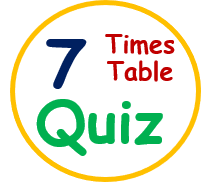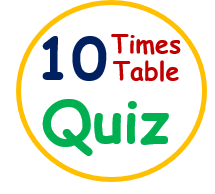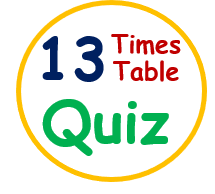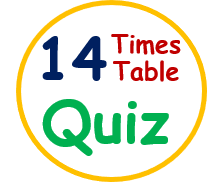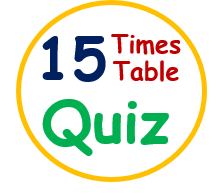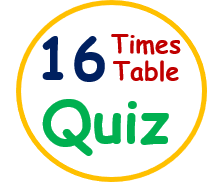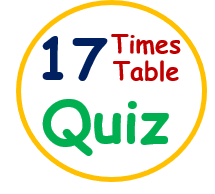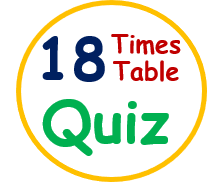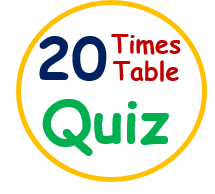If you are looking for some other topics of General knowledge like Animal GK, Solar System GK, Interesting facts about animals and birds, Science GK, Computer GK, Logical maths riddles, General Riddles, World’s Top 10, Sports GK, Inventors, and their invention, Please do visit our YouTube channel by clicking on Shiv Kids Education.

After clicking on the above URL, You will reach to our YouTube channel named “Shiv Kids Education” with a Home page that contains recently added videos. You can access all videos by clicking on the “VIDEOS” tab over there and can also view playlist videos by hitting the “PLAYLIST” tab option.

Enjoy here other topics as well by clicking on the below-given links, We ensure that you would have a wonderful GK Quizzing!